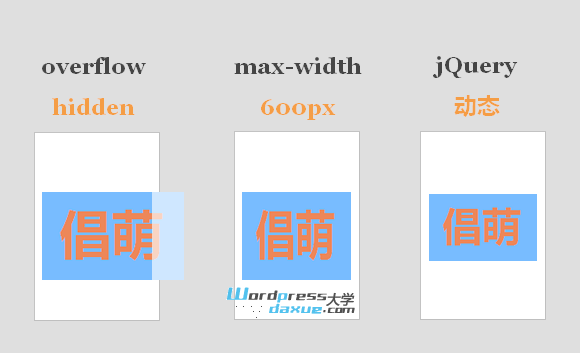WordPress使用jQuery 按比例调整图片高度/宽度

2013-4-14更新：使用 max-width 也是可以按等比缩小图片的。以前倡萌之所以任务无法实现，是因为我自己使用WLW离线发布时，使用WLW定义了图片的格式，而没办法继承样式表的样式。特此说明，希望大家不要被误导！

三种方法的效果比较等比例调整图片的高和宽

方法一：jQuery 代码（荐）

 12345678910111213141516171819202122232425 \$(document).ready(function() { \$('.post img').each(function() { var maxWidth = 600; // 图片最大宽度 var maxHeight = 2000; // 图片最大高度 var ratio = 0; // 缩放比例 var width = \$(this).width(); // 图片实际宽度 var height = \$(this).height(); // 图片实际高度 // 检查图片是否超宽 if(width > maxWidth){ ratio = maxWidth / width; // 计算缩放比例 \$(this).css("width", maxWidth); // 设定实际显示宽度 height = height * ratio; // 计算等比例缩放后的高度 \$(this).css("height", height * ratio); // 设定等比例缩放后的高度 } // 检查图片是否超高 if(height > maxHeight){ ratio = maxHeight / height; // 计算缩放比例 \$(this).css("height", maxHeight); // 设定实际显示高度 width = width * ratio; // 计算等比例缩放后的高度 \$(this).css("width", width * ratio); // 设定等比例缩放后的高度 } });});

方法二：纯Javascript代码

 1234567891011121314151617181920212223242526

WordPress教程排行